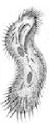Teaching Physics with the Physics Suite Edward F. Redish Problems Sorted by Type | Problems Sorted by Subject | Problems Sorted by Chapter in UP

Propelling a paramecium

 Unicellular organisms such as bacteria and protists are small objects that live in dense fluids. As a result, the resistive force they feel is large and viscous. Since their masses are small their motion looks very different from motion in a medium with little resistance. Paramecia move by pushing their cilia (little hairs on their surface) through the fluid. The fluid (of course) pushes back on them. We will call this back force of the fluid on the cilia of the paramecium "the applied force", F (since it wouldn't happen if the paramecium didn't try to move its cilia). Write Newton's second law for a paramecium feeling two forces: the applied force and the viscous force. (Recall that the viscous force takes the form F viscous = - η v, proportional to the velocity and in the opposite direction.) If the mass is small enough, for most of the time the term "ma" can be much smaller than the two forces, which are large and nearly cancel. Write what the equation for Newton's second law (N2) turns into if we ignore the "ma" term. Describe what the motion would be like and how it would appear different from a low or no resistance example. Suppose the paramecium is starting from rest and starts to move, coming quickly to a constant velocity. Describe how the three terms in the full N2 equation behave, illustrating your discussion with graphs of x, v, a, F net, F, and F viscous.